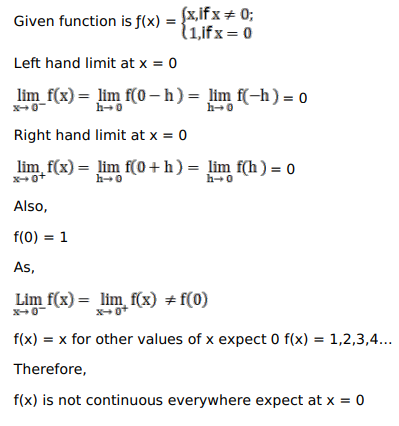# Solve this followingQuestion:

Show that $f(x)=\left\{\begin{array}{ll}x, & \text { if } x \neq 0 ; \\ 1, & \text { if } x=0\end{array}\right.$ is continuous at each point except $0 .$

Solution: